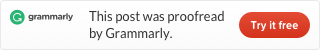# LeetCode 219. Contains Duplicate II

Given an array of integers and an integer k, find out whether there are two distinct indices i and j in the array such that nums[i] = nums[j]and the absolute difference between i and j is at most k.

Example 1:

```Input: nums = [1,2,3,1], k = 3
Output: true
```

Example 2:

```Input: nums = [1,0,1,1], k = 1
Output: true
```

Example 3:

```Input: nums = [1,2,3,1,2,3], k = 2
Output: false```

This problem is similar to 217. Contains Duplicate, but a little harder. First we rule out some conditions (If k==0, must return false. if nums.length<2 must return false. And if k>nums.length we need let k=nums.length.) :

Then, we look at first k elements, if there is a duplicate, then return true. If k==nums.length, but we don’t find any duplicate return false.

Then we start from k+1, build a fixed-width sliding window. Every time we move one postion, we remove the oldest number from the window, add the new number in the window. In this process, if we find a duplicate return true. When all numbers are checked, we still can’t find a duplicate return false.

Want to know details of the sliding window algorithm, check my post: The Sliding Window Algorithm for string and array.

To see more hashtable related problems, see
Problems and Solutions of Hashmap.This site uses Akismet to reduce spam. Learn how your comment data is processed.